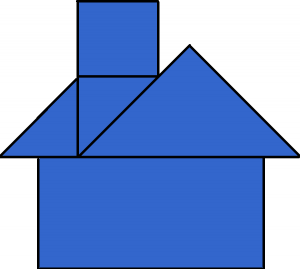# Composite 2D Shapes

Directions: What shapes could be used to create this picture?Make a list of the shapes needed, and how many of each you would need.

### Hint

What shapes can you combine to create the picture?

There can be many different student solutions. One possible answer would be one rectangle, one square and three triangles.Source: Bryan Anderson

## Add Some, Subtract Some

Directions: Use the digits 1-9, at most once, to complete the equation. What is the …

1.I like this question. The answers you get might bring up a lot of good geometric vocabulary depending upon how students define shape. Do the shapes have to be polygons? Do they have to be convex polygons? This house could just be created with one big concave hendecagon.

2.Three triangles,two squares, and a rectangle.

•triangles squares and rectangle

3.triangles, squares, rectangle

4.square, rectangle, triangle

5.i see two triangles one rectangle one square

6.cube, pyramid, triangle

7.8.For this picture we can use a square, triangles and a rectangle.

9.Poorv Dasgupta

Triangle, triangle, rectangle , square makes this picture

10.There are many different combinations. You could also use three rectangles and 3 triangles.

11.Square, Triangle, Rectangle, and a parallagram

•triangles squares and rectangleiyiuti7ti7t7ut757

12.2 triangles, 1 square, 1 rectangle.

13.One square, Two triangles, one rectangle

14.1 rectangle,1 square and 3 triangles